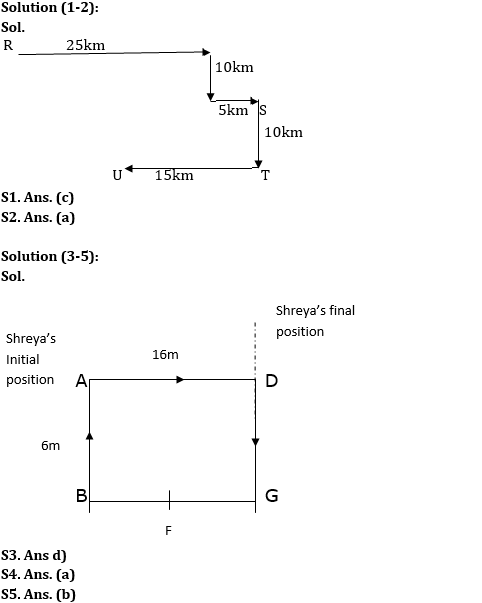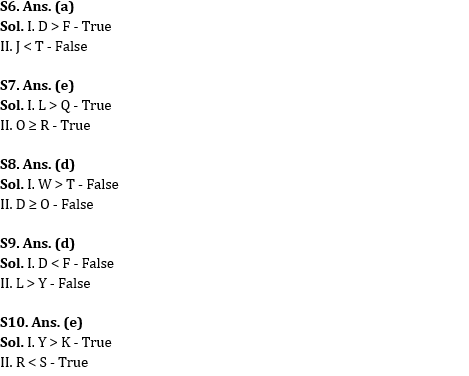Latest Banking jobs   »   NIACL AO reasoning

# Reasoning Quiz For NIACL AO Prelims 2023-01st September

Directions (1-2): Study the information given below and answer the questions based on it:
A person starts his journey from point R. He drives 25 km in east direction and then take a right turn and drives 10km. After taking left turn he reached point S after driving 5 km. Point T is 10 km to the south of S. From T, he is driving 15 km to the west to reach point U. Point S is to the southeast with respect to Point R.

Q1. If point K is 20km to the south of R, then what is the shortest distance between K and U?
(a) 20km
(b) 25km
(c) 15km
(d) 30km
(e) 35km

Q2. What is the direction of point U with respect to point S?
(a) South-west
(b) South-east
(c) East
(d) North-west
(e) None of these

Directions (3-5): Study the information given below and answer the questions based on it:
Point A is 6m to the north of point B. Point D is 16m to the east of point A. Point F is exactly in the middle of B and G. G is 6m south of D. Shreya is standing at point A, she walks 16m towards the east and then turns to her left and walks 6m.

Q3. In which direction is Shreya now with respect to her initial position?
(a) South-west
(b) South-east
(c) East
(d) North-east
(e) None of these

Q4. What is the shortest distance between D and F?
(a) 10m
(b) 15m
(c) 20m
(d) 30m
(e) 35m

Q5. What is the direction of point G with respect to point A?
(a) South-west
(b) South-east
(c) East
(d) North-west
(e) None of these

Directions (6-10): In the following questions assuming the given statement to be true, find which of the conclusion(s) among given conclusions is/are definitely true and then give your answers accordingly.

Q6. Statement: D > L ≥ J ≥ F ≤ U < G ≤ T
Conclusions:
I. D > F
II. J < T
(a) If only conclusion I is true
(b) If only conclusion II is true
(c) If either conclusion I or II is true
(d) If neither conclusion I nor II is true
(e) If both conclusions I and II are true

Q7. Statement: L ≥ C > D ≥ E = R; O ≥ D ≥ Q
Conclusions:
I. L > Q
II. O ≥ R
(a) If only conclusion I is true
(b) If only conclusion II is true
(c) If either conclusion I or II is true
(d) If neither conclusion I nor II is true
(e) If both conclusions I and II are true

Q8. Statements: N < D ≥ W; O ≥ T > G; N > O
Conclusions:
I. W > T
II. D ≥ O
(a) If only conclusion I is true
(b) If only conclusion II is true
(c) If either conclusion I or II is true
(d) If neither conclusion I nor II is true
(e) If both conclusions I and II are true

Q9. Statement: Y < O < D > I = L > F
Conclusions:
I. D < F
II. L > Y
(a) If only conclusion I is true
(b) If only conclusion II is true
(c) If either conclusion I or II is true
(d) If neither conclusion I nor II is true
(e) If both conclusions I and II are true

Q10. Statements: A < K < D > R; Y > D < S
Conclusions:
I. Y > K
II. R < S
(a) If only conclusion I is true
(b) If only conclusion II is true
(c) If either conclusion I or II is true
(d) If neither conclusion I nor II is true
(e) If both conclusions I and II are true

Solutions## FAQs

### What are the subjects asked in NIACL AO Prelims exam 2023?

Subjects asked in NIACL AO Prelims are Reasoning Ability

#### Congratulations!Union Budget 2023-24: Free PDF